Courses

# Worksheet - Long And Short Class 4 Notes | EduRev

## Mathematics (Maths) for Class 4

Created by: Yashina Kapoor

## Class 4 : Worksheet - Long And Short Class 4 Notes | EduRev

The document Worksheet - Long And Short Class 4 Notes | EduRev is a part of the Class 4 Course Mathematics (Maths) for Class 4.
All you need of Class 4 at this link: Class 4

Q.1. Convert to the units shown:
(a) 54 m =____ cm
(b) 55 m = ____ cm
(c) 69 m = ____ cm
(d) 63 m = ____ mm
(e) 53 cm =  ____ mm
(f) 30 m = ____ cm
(g) 47 m = ____ mm
(h) 71 cm = ____ mm
(i) 44 cm = ____ mm
(j) 45 m = ____ cm
(k) 5,000 cm = ____ m
(l) 5,000 mm = ____ cm
(m) 4,000 mm = ____ m
(n) 8,000 mm = ____ cm
(o) 8,000 cm = ____ m
(p) 2,000 mm = ____ m
(q) 9,000 cm = ____ m
(r) 6,000 cm = ____ m
(s) 1,000 mm = ____ cm
(t) 2,000 mm = ____ cm
Ans.

As we know, 1m = 100 cm
1m = 1000 mm
(a) 5,400
(b) 5,500
(c) 6,900
(d) 63,000
(e) 530
(f) 3,000
(g) 47,000
(h) 710
(i) 440
(j) 4,500
(k) 50
(l) 500
(m) 4
(n) 800
(o) 80
(p) 2
(q) 90
(r) 60
(s) 100
(t) 200

Q.2. Circle the longest box.
(a)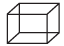(b)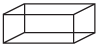(c)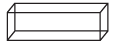(d)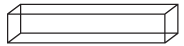Ans.
(d)Q.3. Fill in the blanks
(a) 10 m = ____ cm
(b) 50 m = ____ cm
Ans.

(a) 1000 cm
(b) 5000 cm

Q.4. Complete the table

 Metres ____ 5 7 ____ Centimetres 100 ____ ____ 800

Ans.

 Metres 1 5 7 8 Centimetres 100 500 700 800

Q.5. A room has 6 m length and 4 m breadth. What is the perimeter of the room?
Ans.
20 m

Q.6. Convert into metres
(a) 7 km = ____ m
(b) 2 km 500 = ____ m
(c) 15 km 305 m = ____ m
(d) 9 km 50 m = ____ m
Ans.

(a) 7000 m
(b) 2500 m
(c) 15305 m
(d) 9050 m

Q.7. How much money Renu have if she has 5 (₹ 10 notes) + 3 (₹ 50 notes) + 10 (₹ 100 notes) =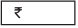Ans.
₹ 1200

Q.8. Measure the shortest distance between point A and B.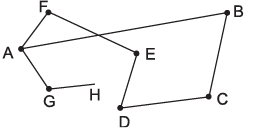Ans.
5.6 cm

Q.9. Find the perimeter of the given figure.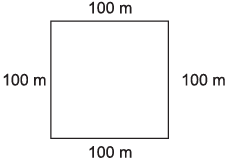Ans.
400 m

Q.10. Ram is 1 m 23 cm tall. Seeta is 12 cm shorter than Ram. What is their total height?
Ans.
2 m 34 cm

Q.11. Find the total distance covered by Mohan from home to school.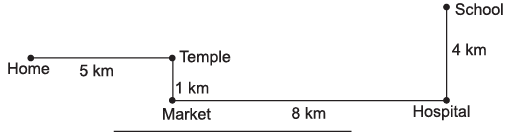Ans.
18 km

Q.12. Which city has the farthest distance point X?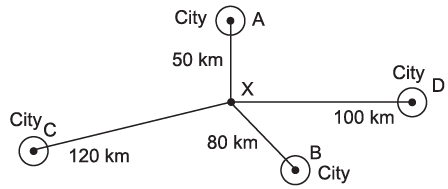(a) City A
(b) City B
(c) City C
(d) City D
Ans.
(c) City C

Q.13. Ram is 150 cm tall and his sister Teena is 115 cm tall. Who is taller and by how many cms?
Ans.
Ram is taller than Seeta by 35 cms.

Q.14. Using a ruler, draw lines of following measurements
(a) 7.7 cm
(b) 6.5 cm
Ans.
Students should draw it themselves.

,

,

,

,

,

,

,

,

,

,

,

,

,

,

,

,

,

,

,

,

,

;